# GRE Subject Test: Math : Continuous Distributions

## Example Questions

← Previous 1 2 Next →

### Example Question #1 : Confidence Intervals & Hypothesis Testing

A sample of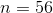observations of 02 consumption by adult western fence lizards gave the following statistics: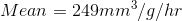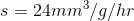Find the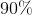confidence limit for the mean 02 consumption by adult western fence lizards.Explanation:

Because we are only given the sample standard deviation we will use the t-distribution to calculate the confidence interval.

Appropriate Formula: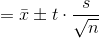Now we must identify our variables: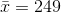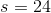We must find the appropriate t-value based on the given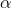t-value at 90% confidence: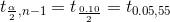Look up t-value for 0.05, 55 , so t-value= ~ 1.6735

90% CI becomes: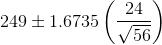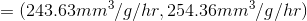### Example Question #1 : Confidence Intervals And Mean

 Subject Horn Length (in) Subject Horn Length (in) 1 19.1 11 11.6 2 14.7 12 18.5 3 10.2 13 28.7 4 16.1 14 15.3 5 13.9 15 13.5 6 12.0 16 7.7 7 20.7 17 17.2 8 8.6 18 19.0 9 24.2 19 20.9 10 17.3 20 21.3

The data above represents measurements of the horn lengths of African water buffalo that were raised on calcium supplements. Construct a 95% confidence interval for the population mean for horn length after supplments.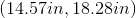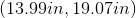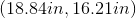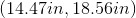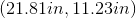Explanation:

First you must calculate the sample mean and sample standard deviation of the sample.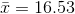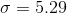Because we do not know the population standard deviation we will use the t-distribution to calculate the confidence intervals. We must use standard error in this formula because we are working with the standard deviation of the sampling distribution.

Formula: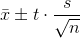To find the appropriate t-value for 95% confidence interval: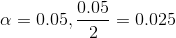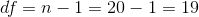Look up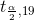in t-table and the corresponding t-value = 2.093.

Thus the 95% confidence interval is: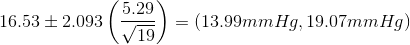### Example Question #1 : Confidence Intervals And Mean

The 95% confidence interval created for the difference in means between two training programs for middle distance college runners is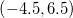. The variable being measured is the improvement in seconds of mile times over the course of a season. One program has more speed work and intervals, while the other focuses more on distance training.

What does the confidence interval tell us about the difference in the two programs?

The mean improvement ofsecond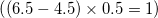is too small to matter, so reject the null. There is no evidence that one program is better at reducing miles times.

The confidence interval is large, so one program is clearly better at reducing mile times than the other.

Zero is in the interval, so do not reject the null. There is no evidence that one program is better than the other.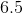is greater than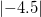, so reject the null. This is evidence that one program is better at reducing mile times than the other.

Zero is not in the interval, so reject the null. This is evidence that one program is significantly better at reducing mile times.

For there to be a statistically significant difference in the training programs, the 95% confidence interval cannot include zero.includes zero, so we can't say that one program is significantly better than the other.